# NEET Physics Electrostatic Potential and Capacitance Questions SolvedAIIMS - 1995

Four equal charges Q are placed at the four corners of a square of each side is ‘a’. Work done in removing a charge – Q from its centre to infinity is

(1) 0

(2) $\frac{\sqrt{2}{Q}^{2}}{4\pi {\epsilon }_{0}a}$

(3) $\frac{\sqrt{2}{Q}^{2}}{\pi {\epsilon }_{0}a}$

(4) $\frac{{Q}^{2}}{2\pi {\epsilon }_{0}a}$

Concept Videos :-

#1 | Defining Electric Potential Energy
#10 | Electric Potential Energy

Concept Questions :-

Electric potential energy

(3) Potential at centre O of the square

${V}_{O}=4\text{\hspace{0.17em}}\left(\frac{Q}{4\pi {\epsilon }_{0}\left(a/\sqrt{2}\right)}\right)$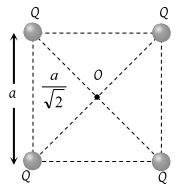Work done in shifting (– Q) charge from centre to infinity $W=-\text{\hspace{0.17em}}Q\left({V}_{\infty }-{V}_{O}\right)=Q{V}_{0}$

$=\frac{4\sqrt{2}\text{\hspace{0.17em}}{Q}^{2}}{4\pi {\epsilon }_{0}a}=\frac{\sqrt{2}{Q}^{2}}{\pi {\epsilon }_{0}a}$

Difficulty Level:

• 48%
• 17%
• 30%
• 7%
Crack NEET with Online Course - Free Trial (Offer Valid Till September 19, 2019)
PMT - 1999

Two spheres of radius a and b respectively are charged and joined by a wire. The ratio of electric field of the spheres is

(1) a/b

(2) b/a

(3) a2/b2

(4) b2/a2

Concept Videos :-

#7 | Relation b/w Electric Field&Potential 1
#8 | Relation b/w Electric Field&Potential 2

Concept Questions :-

Relation between field and potential

(2) Joined by a wire means they are at the same potential. For same potential $\frac{k{Q}_{1}}{{a}_{1}}=\frac{k{Q}_{2}}{{a}_{2}}$

$\frac{{Q}_{1}}{{Q}_{2}}=\frac{a}{b}$

Further, the electric field at the surface of the sphere having radius R and charge Q is $\frac{kQ}{{R}^{2}}.$

$\frac{{E}_{1}}{{E}_{2}}=\frac{k{Q}_{1}/{a}^{2}}{k{Q}_{2}/{b}_{2}}=\frac{{Q}_{1}}{{Q}_{2}}×\frac{{b}^{2}}{{a}^{2}}=\frac{b}{a}$

Difficulty Level:

• 16%
• 34%
• 17%
• 35%
Crack NEET with Online Course - Free Trial (Offer Valid Till September 19, 2019)
PMT - 2000

An electron of mass m and charge e is accelerated from rest through a potential difference V in vacuum. The final speed of the electron will be

(1) $V\sqrt{e/m}$

(2) $\sqrt{eV/m}$

(3) $\sqrt{2eV/m}$

(4) $2eV/m$

Concept Videos :-

#1 | Defining Electric Potential Energy
#10 | Electric Potential Energy

Concept Questions :-

Electric potential energy

(3) Kinetic energy $K=\frac{1}{2}m{v}^{2}=eV$

$v=\sqrt{\frac{2eV}{m}}$

Difficulty Level:

• 7%
• 18%
• 70%
• 7%
Crack NEET with Online Course - Free Trial (Offer Valid Till September 19, 2019)
NEET - 2017

A capacitor is charged by a battery.The battery is removed and another identical uncharged capacitor is connected in parallel. The total electrostatic energy of resulting system

(a)increases by a factor of 4

(b)decreases by a factor of 2

(c)remain the same

(d)increases by a factor of 2

Concept Videos :-

#23 | Combination of Capacitors
#24 | Practise Problems
#32 - Derivation: Combination of Capacitors

Concept Questions :-

Combination of capactiors

(b)Total potential Energy stored in a system of capacitors

=$\Sigma \frac{1}{2}C{V}^{2}$

Also, potential drop remains same in parallel across both capacitors.

Initially stored energy

${U}_{1}=\frac{1}{2}C{V}^{2}$

Finally, potential drop across each capacitor will be  V/2.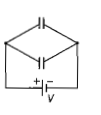So, finally stored energy

${U}_{2}=\frac{1}{2}C{\left(\frac{V}{2}\right)}^{2}+\frac{1}{2}C{\left(\frac{V}{2}\right)}^{2}$

=$\frac{1}{2}\left(2C\right){\left[\frac{V}{2}\right]}^{2}$

=$\left(\frac{1}{4}C{V}^{2}\right)=\frac{{U}_{1}}{2}$

Difficulty Level:

• 12%
• 44%
• 30%
• 16%
Crack NEET with Online Course - Free Trial (Offer Valid Till September 19, 2019)
NEET - 2017

The diagrams below show regions of equipotentials.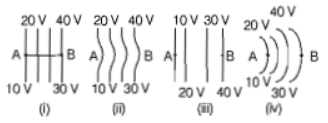A positive charge is moved from A to B in each diagram.

(a) Maximum work is requried to move q in figure(iii)

(b) In all the four cases,the work done is the same

(c) Minimum work is requried to move q in the figure(i)

(d) Maximum work is required to move q in figure(ii)

Concept Videos :-

#9 | Equipotential Surfaces

Concept Questions :-

Equipotential surfaces

(b) We know that, Work done (W)=q $∆V$

$∆V$ is same in all the cases. So, work done will be same in the all cases.

Difficulty Level:

• 9%
• 71%
• 16%
• 6%
Crack NEET with Online Course - Free Trial (Offer Valid Till September 19, 2019)
NEET - 2016

A parallel-plate capacitor of area A, plate separation d and capacitance C is filled with four dielectric materials having dielectric constants and ${k}_{4}$ as shown in the figure below. If a single dielectric material is to be used to have the same capacitance C in this capacitor, then its dielectric constant k is given by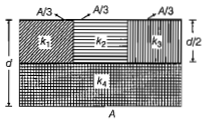(a) $k={k}_{1}+{k}_{2}+{k}_{3}+3{k}_{4}$

(b) $k=\frac{2}{3}\left({k}_{1}+{k}_{2}+{k}_{3}\right)+2{k}_{4}$

(c) $\frac{2}{k}=\frac{3}{{k}_{1}+{k}_{2}+{k}_{3}}+\frac{1}{{k}_{4}}$

(d) $\frac{1}{k}=\frac{1}{{k}_{1}}+\frac{1}{{k}_{2}}+\frac{1}{{k}_{3}}+\frac{3}{2{k}_{4}}$

Concept Videos :-

#23 | Combination of Capacitors
#24 | Practise Problems
#32 - Derivation: Combination of Capacitors

Concept Questions :-

Combination of capactiors

(*) Given capacitor is equivalent to capacitors in parallel and part of ${k}_{4}$ in series with them.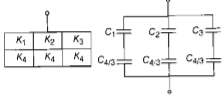No option is matching.

this question is added because it had appeared in NEET exam.

Difficulty Level:

• 17%
• 25%
• 44%
• 16%
Crack NEET with Online Course - Free Trial (Offer Valid Till September 19, 2019)
NEET - 2016

A capacitor of 2$\mu F$ is charged as shown in the figure. When the switch S is turned to position 2, the percentage of its stored energy dissipated is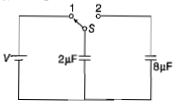(a) 20%               (b) 75%

(c) 80%               (d) 0%

Concept Videos :-

#25 | Energy Stored in Capacitor
#26 | Work Done by Battery & Heat Generated
#27 | Practise Problems
#33 - Derivation: Energy stored in Capacitors

Concept Questions :-

Energy stored in capacitor

(c) Consider the given figure, When the switch S is connected to point 1, then initial energy stored in the capacitor can be given as

=$\frac{1}{2}\left(2\mu F\right)×{V}^{2}$

When the switch S is connected to point 2, energy dissipated on connection across 8$\mu F$ will be

= $\frac{1}{2}\left(\frac{{C}_{1}{C}_{2}}{{C}_{1}+{C}_{2}}\right){V}^{2}$

=

=$\frac{1}{2}×\left(16\mu F\right)×{V}^{2}$

Therefore, % loss of energy =$\frac{16}{2}×100=80%$

Difficulty Level:

• 16%
• 20%
• 47%
• 19%
Crack NEET with Online Course - Free Trial (Offer Valid Till September 19, 2019)
NEET - 2015

A parallel plate air capacitor of capacitance C is connected , to a cell of emf V and then disconnected from it. A dielectric slab of dielectric constant K, which can just fill the air gap of the capacitor, is now inserted in it. Which of the following is incorrect?

(a) The potential difference between the plates decreases K times

(b) The energy stored in the capacitor decreases K times

(c) The change in energy stored is $\frac{1}{2}$CV2($\frac{1}{K}$-1)

(d) The charge on the capacitor is not conserved

Concept Videos :-

#25 | Energy Stored in Capacitor
#26 | Work Done by Battery & Heat Generated
#27 | Practise Problems
#33 - Derivation: Energy stored in Capacitors

Concept Questions :-

Energy stored in capacitor

When a parallel plate air capacitor connected to a coil of emf V, then charge stored will be

q=CV

=> V=q/C

Also energy stored is U=$\frac{1}{2}$CV2=$\frac{{q}^{2}}{2C}$

As the battery is disconnected from the capacitor the charge will not be destroyed i.e. q'=q with the introduction of dielectric in the gap of capacitor the new capacitance will be
C'=CK

=> V'=q/C'=q/CK

The new energy stored  will be

U'=$\frac{{q}^{2}}{2CK}$

ΔU=U'-U=$\frac{{q}^{2}}{2c}$($\frac{1}{K-1}$)

= $\frac{1}{2}$CV2($\frac{1}{K}$-1)

Difficulty Level:

• 16%
• 19%
• 29%
• 38%
Crack NEET with Online Course - Free Trial (Offer Valid Till September 19, 2019)
NEET - 2015

If potential (in volts) in a region is expressed as V(x,y,z)=6xy-y+2yz, the electric field (in N/C) at point (1,1,0) is

(a)-(3$\stackrel{^}{i}$+5$\stackrel{^}{j}$+3$\stackrel{^}{\mathrm{k}}$)
(b)-(6$\stackrel{^}{i}$+5$\stackrel{^}{j}$+2$\stackrel{^}{\mathrm{k}}$)
(c)-(2$\stackrel{^}{i}$+3$\stackrel{^}{j}$+$\stackrel{^}{\mathrm{k}}$)
(d)-(6$\stackrel{^}{i}$+9$\stackrel{^}{j}$+$\stackrel{^}{\mathrm{k}}$

Concept Videos :-

#7 | Relation b/w Electric Field&Potential 1
#8 | Relation b/w Electric Field&Potential 2

Concept Questions :-

Relation between field and potential

Given, potential in a region V=6xy-y+2yz

Electric field in a region

E=$\partial$V/$\partial$$\stackrel{^}{i}$ $\partial$V/$\partial$y -$\stackrel{^}{j}$ $\partial$V/$\partial$$\stackrel{^}{\mathrm{k}}$

=> E=-6y$\stackrel{^}{i}$-(6x-1)$\stackrel{^}{j}$-2y$\stackrel{^}{\mathrm{k}}$

at (1,1,0) electric field can be expressed

E=-(6 X 1)$\stackrel{^}{i}$-(6 x 1-1)$\stackrel{^}{j}$-2 x 1$\stackrel{^}{\mathrm{k}}$

=-(6$\stackrel{^}{i}$+5$\stackrel{^}{j}$+2$\stackrel{^}{\mathrm{k}}$) N/C

Difficulty Level:

• 9%
• 69%
• 14%
• 11%
Crack NEET with Online Course - Free Trial (Offer Valid Till September 19, 2019)
NEET - 2015

A parallel plate air capacitor has capacity C, distance of separation between plates is d and potential difference V is applied between the plates. Force of attraction between the plates of the parallel plate air capacitor is

(a)C2V2/2d

(b)CV2/2d

(c)CV2/d

(d)C2V2/2d2

Force between plates of parallel capacitor

F=qE=q[$\sigma$/2$\epsilon$c]

∴Surface charge density $\sigma$=q/A

∴F=q[q/2A$\epsilon$o]

=>F=q2/2A$\epsilon$o

So, net charge across a capacitor, q=CV

F=$\frac{{C}^{2}{V}^{2}}{2{A}^{{\epsilon }_{0}}}$      $\left[c=\frac{A{\epsilon }_{0}}{d}\right]$

=>$F=\left(\frac{A{\epsilon }_{0}}{d}\right)$  x $\frac{C{V}^{2}}{2{A}^{{\epsilon }_{0}}}$

$=\frac{C{V}^{2}}{2d}$

Difficulty Level:

• 14%
• 48%
• 30%
• 10%
Crack NEET with Online Course - Free Trial (Offer Valid Till September 19, 2019)